# Python | Implementation of Polynomial Regression

• Difficulty Level : Medium
• Last Updated : 24 Aug, 2022

Polynomial Regression is a form of linear regression in which the relationship between the independent variable x and dependent variable y is modeled as an nth degree polynomial. Polynomial regression fits a nonlinear relationship between the value of x and the corresponding conditional mean of y, denoted E(y |x)
Why Polynomial Regression:

• There are some relationships that a researcher will hypothesize is curvilinear. Clearly, such types of cases will include a polynomial term.
• Inspection of residuals. If we try to fit a linear model to curved data, a scatter plot of residuals (Y-axis) on the predictor (X-axis) will have patches of many positive residuals in the middle. Hence in such a situation, it is not appropriate.
• An assumption in usual multiple linear regression analysis is that all the independent variables are independent. In polynomial regression model, this assumption is not satisfied.

Uses of Polynomial Regression:
These are basically used to define or describe non-linear phenomena such as:

• The growth rate of tissues.
• Progression of disease epidemics
• Distribution of carbon isotopes in lake sediments

The basic goal of regression analysis is to model the expected value of a dependent variable y in terms of the value of an independent variable x. In simple regression, we used the following equation –

`y = a + bx + e`

Here y is a dependent variable, a is the y-intercept, b is the slope and e is the error rate.
In many cases, this linear model will not work out For example if we analyzing the production of chemical synthesis in terms of temperature at which the synthesis take place in such cases we use a quadratic model

`y = a + b1x + b2^2 + e`

Here y is the dependent variable on x, a is the y-intercept and e is the error rate.
In general, we can model it for nth value.

`y = a + b1x + b2x^2 +....+ bnx^n`

Since regression function is linear in terms of unknown variables, hence these models are linear from the point of estimation.
Hence through the Least Square technique, let’s compute the response value that is y.
Polynomial Regression in Python:
To get the Dataset used for the analysis of Polynomial Regression, click here.
Step 1: Import libraries and dataset
Import the important libraries and the dataset we are using to perform Polynomial Regression.

## Python3

 `# Importing the libraries``import` `numpy as np``import` `matplotlib.pyplot as plt``import` `pandas as pd`` ` `# Importing the dataset``datas ``=` `pd.read_csv(``'data.csv'``)``datas`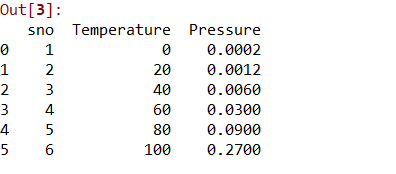Step 2: Dividing the dataset into 2 components
Divide dataset into two components that is X and y.X will contain the Column between 1 and 2. y will contain the 2 columns.

## Python3

 `X ``=` `datas.iloc[:, ``1``:``2``].values``y ``=` `datas.iloc[:, ``2``].values`

Step 3: Fitting Linear Regression to the dataset
Fitting the linear Regression model On two components.

## Python3

 `# Fitting Linear Regression to the dataset``from` `sklearn.linear_model ``import` `LinearRegression``lin ``=` `LinearRegression()`` ` `lin.fit(X, y)`Step 4: Fitting Polynomial Regression to the dataset
Fitting the Polynomial Regression model on two components X and y.

## Python3

 `# Fitting Polynomial Regression to the dataset``from` `sklearn.preprocessing ``import` `PolynomialFeatures`` ` `poly ``=` `PolynomialFeatures(degree ``=` `4``)``X_poly ``=` `poly.fit_transform(X)`` ` `poly.fit(X_poly, y)``lin2 ``=` `LinearRegression()``lin2.fit(X_poly, y)`

Step 5: In this step, we are Visualising the Linear Regression results using a scatter plot.

## Python3

 `# Visualising the Linear Regression results``plt.scatter(X, y, color ``=` `'blue'``)`` ` `plt.plot(X, lin.predict(X), color ``=` `'red'``)``plt.title(``'Linear Regression'``)``plt.xlabel(``'Temperature'``)``plt.ylabel(``'Pressure'``)`` ` `plt.show()`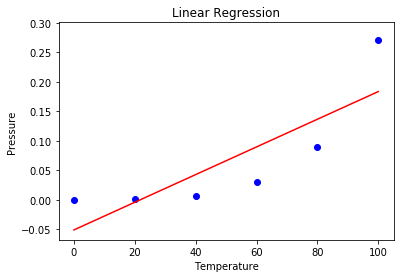Step 6: Visualising the Polynomial Regression results using a scatter plot.

## Python3

 `# Visualising the Polynomial Regression results``plt.scatter(X, y, color ``=` `'blue'``)`` ` `plt.plot(X, lin2.predict(poly.fit_transform(X)), color ``=` `'red'``)``plt.title(``'Polynomial Regression'``)``plt.xlabel(``'Temperature'``)``plt.ylabel(``'Pressure'``)`` ` `plt.show()`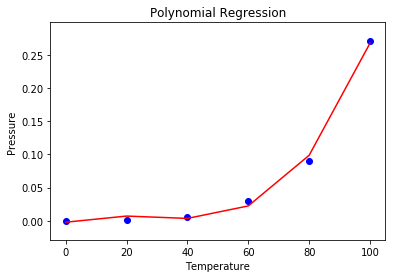Step 7: Predicting new results with both Linear and Polynomial Regression. Note that the input variable must be in a numpy 2D array.

## Python3

 `# Predicting a new result with Linear Regression after converting predict variable to 2D array``pred ``=` `110.0``predarray ``=` `np.array([[pred]])``lin.predict(predarray)`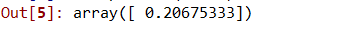## Python3

 `# Predicting a new result with Polynomial Regression after converting predict variable to 2D array``pred2 ``=` `110.0``pred2array ``=` `np.array([[pred2]])``lin2.predict(poly.fit_transform(pred2array))`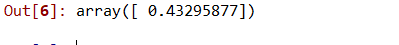Advantages of using Polynomial Regression:

• A broad range of functions can be fit under it.
• Polynomial basically fits a wide range of curvatures.
• Polynomial provides the best approximation of the relationship between dependent and independent variables.

Disadvantages of using Polynomial Regression

• These are too sensitive to the outliers.
• The presence of one or two outliers in the data can seriously affect the results of nonlinear analysis.
• In addition, there are unfortunately fewer model validation tools for the detection of outliers in nonlinear regression than there are for linear regression.

My Personal Notes arrow_drop_up